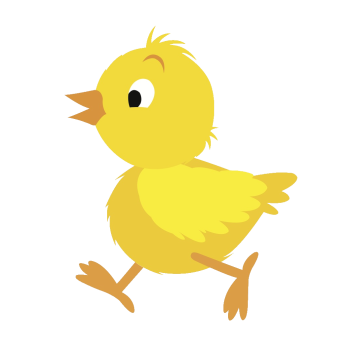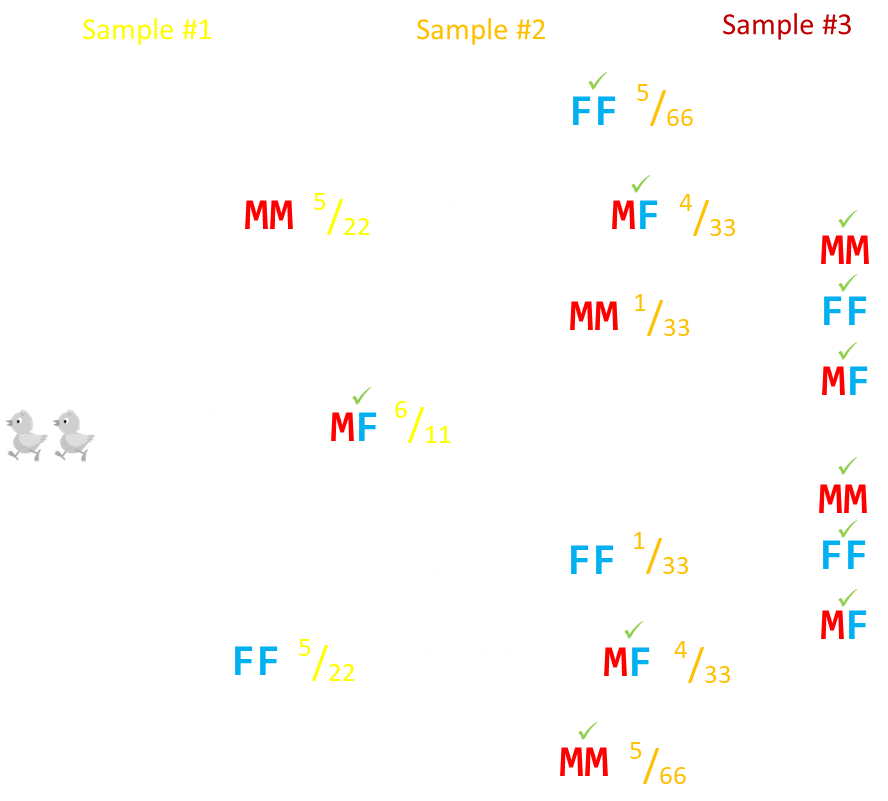# Gender Identification Puzzle [Update]

Yesterday I posted a puzzle about how to select two chicks of opposite gender from a dozen chicks (six of each) using a series of queries.
I gave a solution that would guarantee a solution in exactly two moves; never more, never less. It minimized the maximum regret.
Shortly after publishing, I was contacted by one of my readers Andrew Buchanan who pointed out that it’s possible to come up with a solution with an expected value of less than two queries. (The expected value is the number of moves it would take, on average, to find the solution if we were to repeat the exercise an infinite number of times).
His solution sometimes solves the problem in one move, sometime in two and, in the worst case, three moves. However, there is asymmetry in the chances of each of these paths occurring such that the chance of requiring three moves very small (and there is a reasonable chance of it only requiring one move). This moves the expected value lower than two.
Overall, if repeated lots of times, the expected number of required queries using his strategy is 50/33 or approximately 1.515 times.## Optimal Expected Value Solution

To do better than an expected answer of two, we have to be able to give the picker the chance to solve on the first move. The key to this strategy is the pull the chicks out in pairs.
The first move, therefore, is to pick just two chicks. There’s a chance we get lucky on the first pick; if so, great, we’ve solved in just one move.
If there is not one of each, then the gender of both chicks in the first sample must be the same, either {MM, FF}.
If this happens, we pull two new chicks. Again, there is a chance, in this second pull, to get the chiral pair which is an instant success (two moves), but there is another outcome that allows for success. If the two chicks in the second pull are of the opposite gender to that of the first pull we can also stop (taking one chick from the first sample, and one from the second). The only reason we will need a third sample is if all four of the already pulled chicks are the same gender.
If we require a third pull, we do this by select another two chicks from the cage. We never need more than three samples. If the third sample is one of each, we’ve got what we want. If the third pair match the gender of the first four pulled we’ve identified all the chickens (all the ones remaining in the cage are the opposite gender and we can pull any check from the samples and any from the cage), and if they are opposite we can pick one from the third batch, and any one of the first four.
Another advantage of this solution is that it does not require any chick to be sexed more than once.

## Math

Here are all the possibilities enumerate on a stick diagram.You can see the simple probabilities of going down each path and the probability of getting to each node. Conditions that are successful are marked with a green tick mark.
1. The probability of success in one sample is 6/11.
2. The probability of success in two samples is (5/66 + 4/33 + 5/66 + 4/33) = 13/33.
3. The probability of success in three samples is (1/33 + 1/33) = 2/33.

## Expected value

The expected value is:
[1 x 6/11] + [2 x 13/33] + [3 x 2/33] = 50/33 = 1 17/33